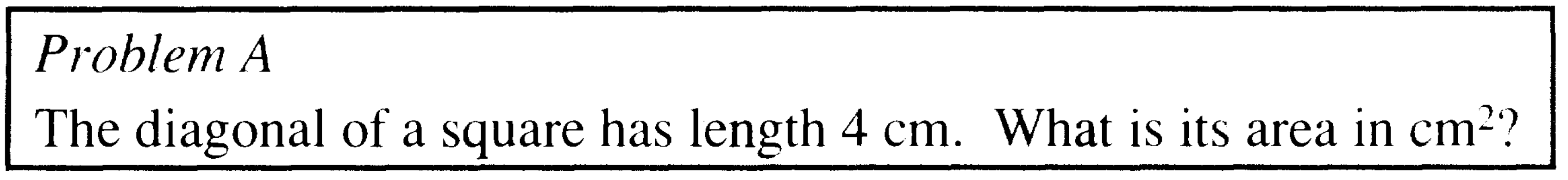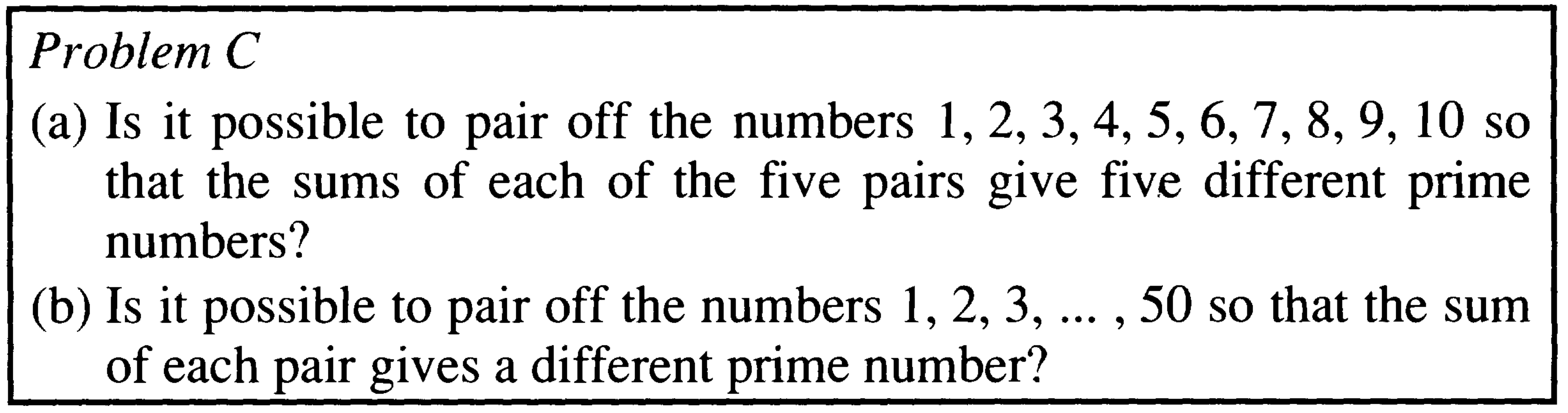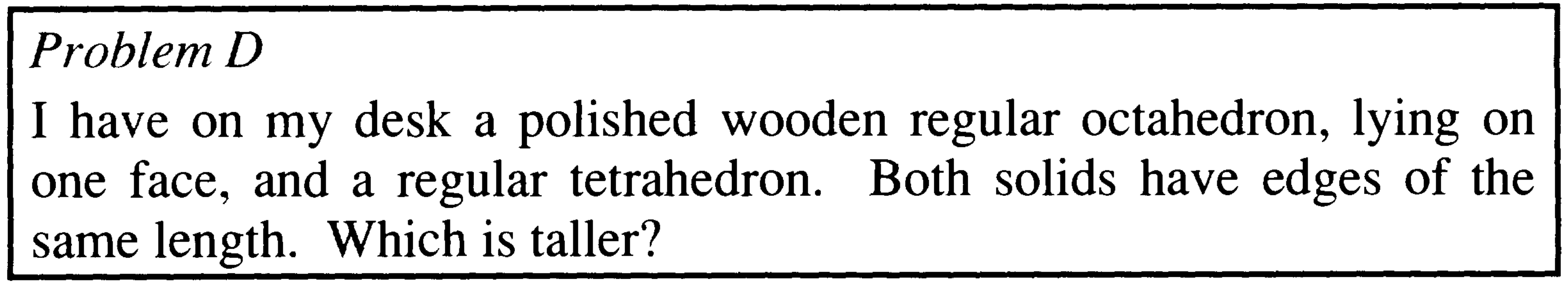# NotCH 4: Five Problems From Tony Gardiner

We have written about Tony Gardiner and excerpted from his writings a number of times: here, here, here, here and here. We will post an entire article by Tony in a day or so, but we are first posting the problems contained within the article. Unlike the “problem” here, the problems below are genuinely presented by Tony to puzzle over, and are only loosely tied to the text of the article. Have fun.## 12 Replies to “NotCH 4: Five Problems From Tony Gardiner”

1.Red Five says:

These are brilliant. Love them.

Still thinking about C part (b) and E.

Won’t post my answers to the other problems; I think problem D is pretty clever for a few reasons.

1.Anonymous says:

C(b): No. There are 25 pairs and the maximum sum of a pair is 50+49=99. There are only 25 primes less than 99 and one of them is 2, which is unobtainable.
E: {8,9,10,12}

1.marty says:

Thanks. Can you solve C(a)?

1.Red Five says:

That (C(a)) wasn’t as difficult as it first appeared. Will hold off posting my solution to allow others to ponder.

E has me thinking still – yes there exists a set of 4 such numbers, but 5 or 6…? Or a different set of 4…?

I feel I’m missing something though… and I love it!

D I think would have been difficult if I hadn’t thought of the answer straight away by considering two tetrahedrons stuck together.

1.marty says:

Yes, E seems to me very interesting.

2.Damo says:

1664, 1665, 1666, 1668, 1680
174101504, 174101505, 174101506, 174101508, 174101520, 174101760

There may be sets that contain smaller integers, but I think these work…

1.marty says:

Thanks, Damo. Do you want to say how you found them?

1.Damo says:

It may be a bit hard to put into a chat, but the vibe is… I decided that I wanted to try and continue the sequence if possible – so, start with three consecutive numbers, then a gap of two. I made a few observations that needed to be true for this to work, such as: the first number had to be a multiple of 4, the second a multiple of 3, the fourth (and any subsequent) number has to be a multiple of 4. I then focused on the differences between pairs of numbers – in particular the first and last number in the sequence. Because these would both be multiples of 4, I focused on the difference between them being a multiple of 4 and used this as a starting point.

I then made lists showing the difference between the last number in the sequence and the others. For example: 8-7-6-4 means a difference of: 8 between the last and the first numbers; 7 between the last and the second, etc. For this list, it would mean a difference (and thus common factor) of 6 between the last and the third number, which would mean that the third would be a factor of three. But the first three numbers are consecutive and the second is a factor of three, so this list was a bust. Similarly 12-11-10-8. But 16-15-14-12 was not. 1680 was the lowest common multiple of these numbers, subtracting the 16,15,14 and 12 gives the first list above.

I think that this approach can be expanded to give a list of six numbers – I thought that I had done it, but checked it again this evening and realised that the third and fifth numbers have a difference of 14, but 14 is not a common factor. I believe all the others worked, so that was a bummer. I’m pretty sure I know where I went wrong and that I could fairly quickly find a correct solution, but I may park it for a while and let others give it a shot. There is still a fair bit of meat to the question: I think I understand how to solve it, but I’ve got a fair bit of work to do to satisfy myself as to why my approach works (or why it would/would not keep working), let alone if my solution would be the simplest (in terms of smallest integers) or if there are other possible sets that don’t start with three consecutive numbers, for example. It’s a pretty rough sketch of my approach, hopefully people can follow.

2.Red Five says:

Impressive. Very impressive.

3.Damo says:

I thought that I would let this lie for a while, but nope. Here is a set of six numbers. The numbers are large. I suspect there is a set of smaller integers that works, but after my last attempt, which fell just short, I am at least confident that this one does work.

The set of numbers will have the following differences between the first number in the set and the subsequent numbers: 1, 2, 4, 16, 65536
Note that for this to work the first (and therefore last) numbers in the set must be multiples of 65536. All of the differences are factors of 65536, and so will be the greatest common factor of the two respective numbers.

The differences between the second number in the set and the subsequent numbers will be: 1, 3, 15, 65535
Note the second (and therefore last) numbers in the set must be multiples of 65535. All of the differences are factors of 65535, and so will be the greatest common factor of the two respective numbers.

The differences between the third number in the set and the subsequent numbers: 2, 14, 65534
Note the third (and therefore last) numbers in the set must be multiples of 65534. All of the differences are factors of 65534, and so will be the greatest common factor of the two respective numbers.

The differences between the fourth number in the set and the subsequent numbers: 12, 65532
Note that the fourth (and therefore last) numbers in the set must be multiples of 65532. Both these differences are factors of 65532, and so will be the greatest common factor of the two respective numbers.

The difference between the fifth and the last number will be 65520. Both numbers must be multiples of 65520.

The prime factorisations of the differences between the last number in the set and each of the other numbers are:
65536=2^16
65535=3*5*17*257
65534=2*7*31*151
65532=2^2*3*43*127
65520=2^4*3^2*5*7*13

Let the largest number in the set be X. We saw above that this number must be a multiple of all of the numbers above. Let it be the lowest common multiple.

X=2^16*3^2*5*7*13*17*31*43*127*151*257

The set is {X – 65536, X – 65535, X – 65534, X – 65532, X – 65520, X}

For what it’s worth, I make this out to be:
{29972757439627526144, 29972757439627526145, 29972757439627526146, 29972757439627526148, 29972757439627526160, 29972757439627591680}

2.Steve R says:

Marti,

Nice puzzles. My favourite from year 7 was identify the counterfeit coin in a dozen identical coins given a traditional two pan balance and 3 weighings and also whether the fake was heavier or lighter than the rest .

PS perhaps now is the time to share some of Martin Gardner’s articles in Scientific American from the 70s a sample of NOTCHES are here https://static.scientificamerican.com/sciam/assets/media/pdf/Aug2008_Martin_Gardner_Recreational_Mathematics.pdf

Steve R

3.Red Five says:

Puzzle A:Puzzle B:Puzzle C(a): Yes.